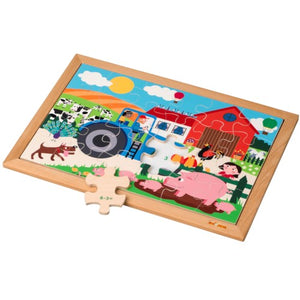# Math puzzle - addition and subtraction up to 6 (24)

Vendor
Educo
Regular price
R 388.48
Sale price
R 388.48
Regular price
R 388.48
Sold out
Unit price
per
Tax included. Shipping calculated at checkout.

Practice the understanding of the numbers 0 to 6. Practice simple addition and subtraction equations that result in a maximum of 6. This wooden arithmetic puzzle contains 24 pieces. Each piece of the puzzle shows subtraction sum or an addition sum. The result to each sum corresponds to a number on the frame. Also note the colour, as some quantities occur more than once.

On the picture itself there is also plenty to see and plenty to count. It's all about the farm. Which animals do you see?  How many cows do you count?  What is flying in the sky?

So in addition to the fun of putting it together, this puzzle promotes the understanding of the numbers 0 to 6, it practices adding and subtracting the numbers 0 to 6, and it improves the vocabulary.

Contents:
wooden frame (40 x 28 cm)
24 wooden puzzle pieces with addition and subtraction equations up to 6